Question

Part A

What volume of 1.00 M HCl in liters is needed to react completely (with nothing left over) with 0.250 L of 0.500 M Na2CO3?

Express your answer to three significant figures and include the appropriate units.

Part B

A 419-mL sample of unknown HCl solution reacts completely with Na2CO3 to form 12.1 g CO2. What was the concentration of the HCl solution? Express your answer to three significant figures and include the appropriate units.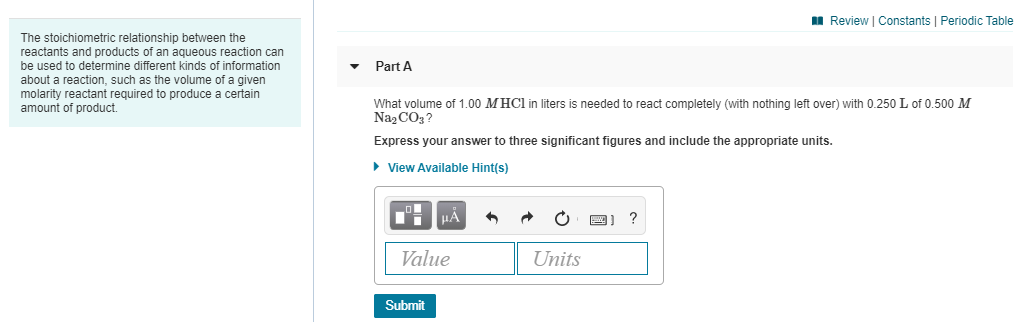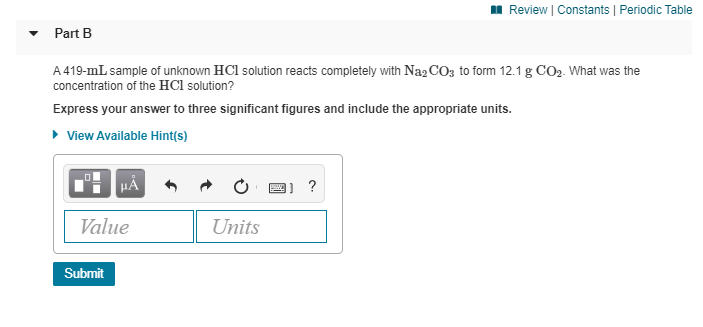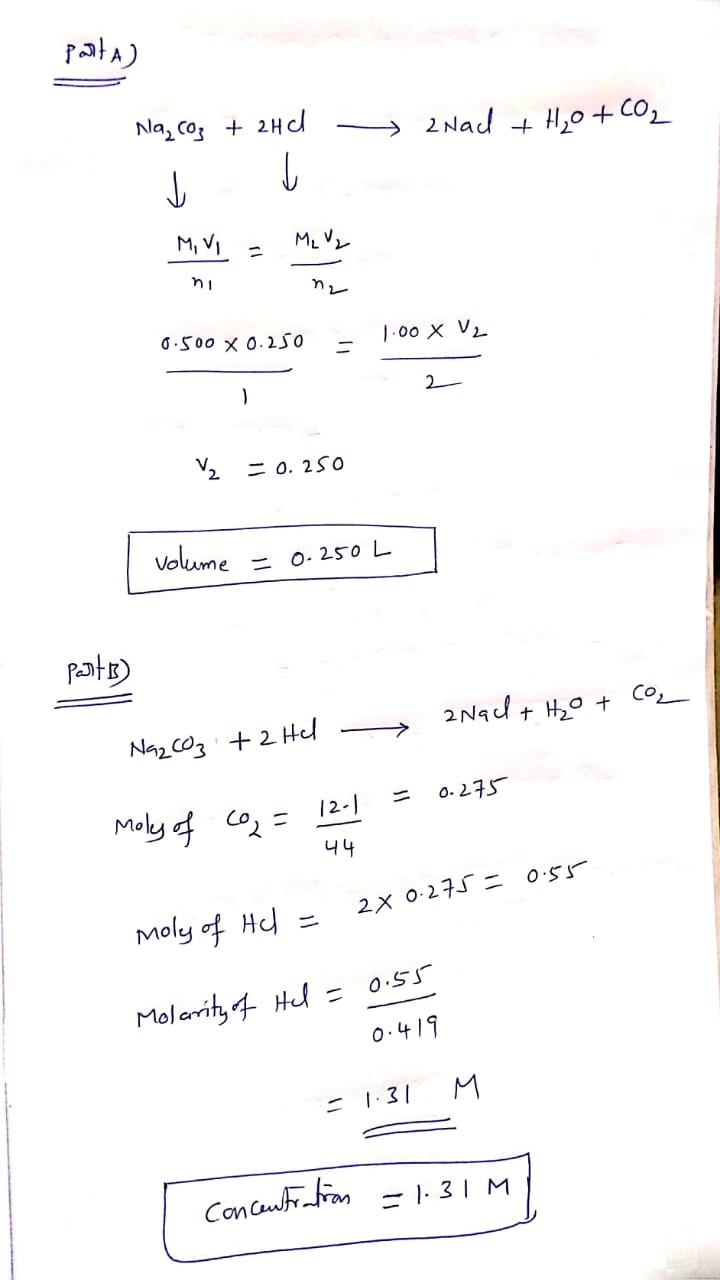#### Earn Coins

Coins can be redeemed for fabulous gifts.

Similar Homework Help Questions
• ### 1. What volume of 1.00 M HCl in liters is needed to react completely (with nothing...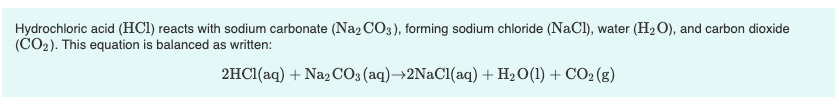1. What volume of 1.00 M HCl in liters is needed to react completely (with nothing left over) with 0.750 L of 0.200 M Na2CO3? Express your answer to three significant figures and include the appropriate units. 2. A 639-mL sample of unknown HCl solution reacts completely with Na2CO3 to form 15.1 g CO2. What was the concentration of the HCl solution? Express your answer to three significant figures and include the appropriate units. Hydrochloric acid (HCl) reacts with sodium...

• ### Part A What volume of 1.75 M HCl in liters is needed to react completely (with...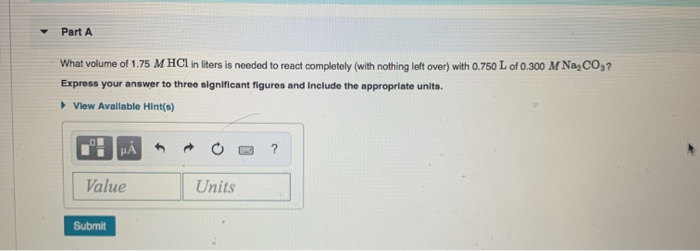Part A What volume of 1.75 M HCl in liters is needed to react completely (with nothing left over) with 0.750 L of 0.300 M NaCO3? Express your answer to three significant figures and include the appropriate units. View Available Hint(s) I HẢ * * 0 -3 ? Value Units Submit

• ### 1. What is the final volume in milliliters when 0.653 L of a 45.4 % (m/v)...

1. What is the final volume in milliliters when 0.653 L of a 45.4 % (m/v) solution is diluted to 24.0 % (m/v)? Express your answer with the appropriate units. 2. A 751 mL NaCl solution is diluted to a volume of 1.06 L and a concentration of 8.00 M . What was the initial concentration? Express your answer with the appropriate units. 3. What volume of 1.00 M HCl in liters is needed to react completely (with nothing left...

• ### 9 of 28 A Review Constants | Periodic Table 2HCl(aq) + Na2CO3(aq) +2NaCl(aq) + H2O(l) +...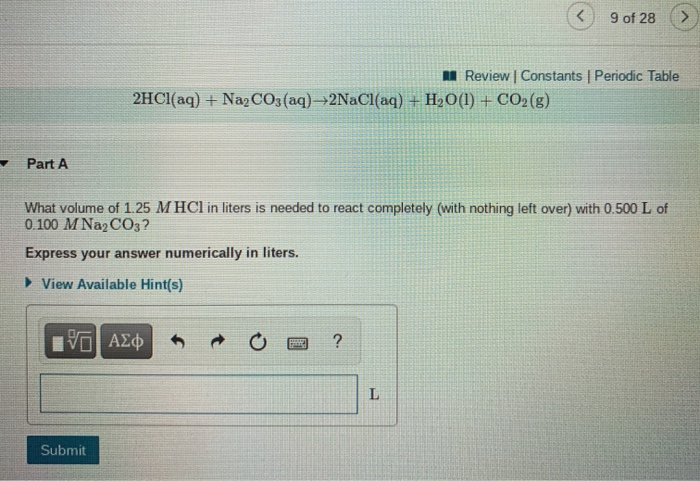9 of 28 A Review Constants | Periodic Table 2HCl(aq) + Na2CO3(aq) +2NaCl(aq) + H2O(l) + CO2(g) Part A What volume of 1.25 M HCl in liters is needed to react completely (with nothing left over) with 0.500 L of 0.100 M Na2CO3? Express your answer numerically in liters. ► View Available Hint(s) ΟΙ ΑΣΦ ? L Submit 9 of 28 Review | Constants Periodic Table Part B A 381-mL sample of unknown HCl solution reacts completely with Na2CO3 to...

• ### The stoichiometric relationship between the reactants and products of an aqueous reaction can be used to...

The stoichiometric relationship between the reactants and products of an aqueous reaction can be used to determine different kinds of information about a reaction, such as the volume of a given molarity reactant required to produce a certain amount of product. Hydrochloric acid (HCl) reacts with sodium carbonate (Na2CO3), forming sodium chloride (NaCl), water (H2O), and carbon dioxide (CO2). This equation is balanced as written: 2HCl(aq)+Na2CO3(aq)→2NaCl(aq)+H2O(l)+CO2(g) a. What volume of 2.50 M HCl in liters is needed to react completely...

• ### 4. The stoichiometric relationship between the reactants and products of an aqueous reaction can be used...

4. The stoichiometric relationship between the reactants and products of an aqueous reaction can be used to determine different kinds of information about a reaction, such as the volume of a given molarity reactant required to produce a certain amount of product. Hydrochloric acid (HCl) reacts with sodium carbonate (Na2CO3), forming sodium chloride (NaCl), water (H2O), and carbon dioxide (CO2). This equation is balanced as written: 2HCl(aq)+Na2CO3(aq)→2NaCl(aq)+H2O(l)+CO2(g) A.) What volume of 3.00 M HCl in liters is needed to react...

• ### The stoichiometric relationship between the reactants and products of an aqueous reaction can be used to...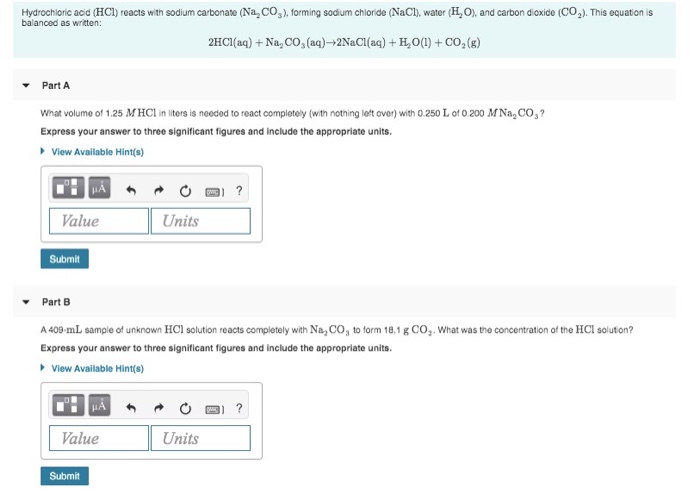The stoichiometric relationship between the reactants and products of an aqueous reaction can be used to determine different kinds of information about a reaction, such as the volume of a given molarity reactant required to produce a certain amount of product. Hydrochloric acid (HCl) reacts with sodium carbonate (Na CO2). forming sodium chloride (NaCl), water (HO), and carbon dioxide (C0.). This equation is balanced as written: 2HCl(aq) + Na, CO, (aq)-2NaCl(aq) + H,0(1) + CO2(g) Part A What volume of...

• ### What volume, in liters, of 1.00 M KOH solution is needed to react completely with 0.100...

What volume, in liters, of 1.00 M KOH solution is needed to react completely with 0.100 L of a 3.00 M H2SO4 solution according to the following equation? 2KOH + H2SO4 → K2SO4 + 2H2O

• ### ASAP What volume, in liters, of 1.00 M Pb(NO3)2 is needed to react completely with 0.500...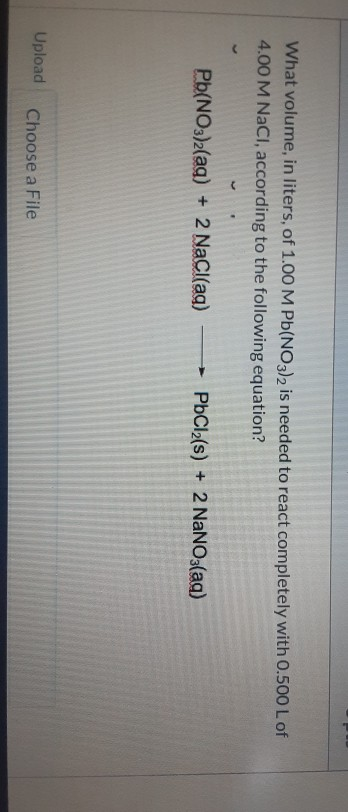ASAP What volume, in liters, of 1.00 M Pb(NO3)2 is needed to react completely with 0.500 L of 4.00 M NaCl, according to the following equation? Pb(NO3)2(aq) + 2 NaCl(ag) – PbCl2(s) + 2 NaNO3(aq) Upload Choose a File

• ### Part B A 457-mL sample of unknown HCl solution reacts completely with Na,COto form 11.1 g...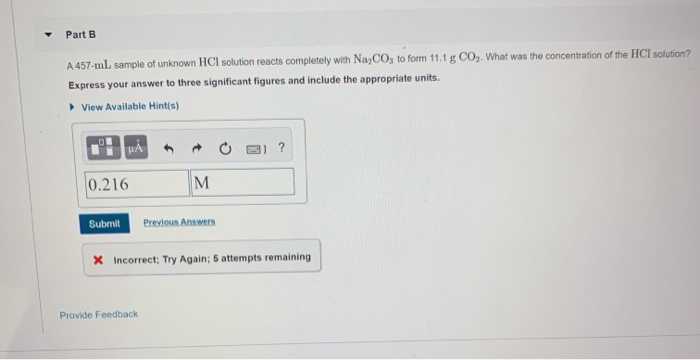Part B A 457-mL sample of unknown HCl solution reacts completely with Na,COto form 11.1 g CO,. What was the concentration of the HCI solution? Express your answer to three significant figures and include the appropriate units View Available Hints) ? .! HAO 110.216 M Submit Previous Answers X Incorrect; Try Again; 5 attempts remaining Provide Feedback